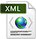New Software and Platforms
Bilateral Contracts and Grants with Industry
Partnerships and Cooperations
BibliographyPDF e-Pub

## Section: Research Program

### Background on non-standard analysis

Non-Standard analysis plays a central role in our research on hybrid systems modeling  , , , . The following text provides a brief summary of this theory and gives some hints on its usefulness in the context of hybrid systems modeling. This presentation is based on our paper , a chapter of Simon Bliudze's PhD thesis  , and a recent presentation of non-standard analysis, not axiomatic in style, due to the mathematician Lindström  .

Non-standard numbers allowed us to reconsider the semantics of hybrid systems and propose a radical alternative to the super-dense time semantics developed by Edward Lee and his team as part of the Ptolemy II project, where cascades of successive instants can occur in zero time by using ${ℝ}_{+}×ℕ$ as a time index. In the non-standard semantics, the time index is defined as a set $𝕋=\left\{n\partial \mid n\in {}^{*}ℕ\right\}$, where $\partial$ is an infinitesimal and ${}^{*}ℕ$ is the set of non-standard integers. Remark that (1) $𝕋$ is dense in ${ℝ}_{+}$, making it “continuous”, and (2) every $t\in 𝕋$ has a predecessor in $𝕋$ and a successor in $𝕋$, making it “discrete”. Although it is not effective from a computability point of view, the non-standard semantics provides a framework that is familiar to the computer scientist and at the same time efficient as a symbolic abstraction. This makes it an excellent candidate for the development of provably correct compilation schemes and type systems for hybrid systems modeling languages.

Non-standard analysis was proposed by Abraham Robinson in the 1960s to allow the explicit manipulation of “infinitesimals” in analysis  , , . Robinson's approach is axiomatic; he proposes adding three new axioms to the basic Zermelo-Fraenkel (ZFC) framework. There has been much debate in the mathematical community as to whether it is worth considering non-standard analysis instead of staying with the traditional one. We do not enter this debate. The important thing for us is that non-standard analysis allows the use of the non-standard discretization of continuous dynamics “as if” it was operational.

Not surprisingly, such an idea is quite ancient. Iwasaki et al.   first proposed using non-standard analysis to discuss the nature of time in hybrid systems. Bliudze and Krob  ,  have also used non-standard analysis as a mathematical support for defining a system theory for hybrid systems. They discuss in detail the notion of “system” and investigate computability issues. The formalization they propose closely follows that of Turing machines, with a memory tape and a control mechanism.